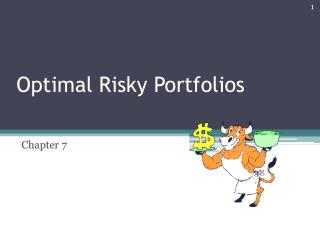# Optimal Risky Portfolios - PowerPoint PPT PresentationDownload PresentationOptimal Risky Portfolios

Optimal Risky PortfoliosDownload Presentation## Optimal Risky Portfolios

- - - - - - - - - - - - - - - - - - - - - - - - - - - E N D - - - - - - - - - - - - - - - - - - - - - - - - - - -
##### Presentation Transcript

1. Optimal Risky Portfolios Chapter 7 Bodi Kane Marcus Ch 5

2. p(%) 35 Unique Risk (unsystematic risk) 20 0 Total Risk Market Risk (systematic risk) 10 20 30 40 1,000+ Number Stocks in Portfolio

3. Portfolios of two risky assets

4. Portfolios of two risky assets =0.19 =19%

5. Bodi Kane Marcus Ch 5 Covariance • A measure of the degree to which returns on two risky assets move in tandem. A positive covariance means that asset returns move together. A negative covariance means returns move inversely.

6. Bodi Kane Marcus Ch 5 Correlation Coefficient A measure that determines the degree to which two variable's movements are associated.The correlation coefficient is calculated as:

7. Bodi Kane Marcus Ch 5 Portfolios of two risky assets • If DE = 1

8. Bodi Kane Marcus Ch 5 Portfolio Expected Return as a function of investment proportion Expected Return, 13% Equity Fund 8% Debt Fund

9. Bodi Kane Marcus Ch 5 Problem 5 Expected Return, 20% Equity Fund 12% Debt Fund

10. Bodi Kane Marcus Ch 5 Portfolio Expected Return as a function of standard deviation  =-1 E  =.30 Expected Return, rp  =0  =1 D Risk, p

11. Bodi Kane Marcus Ch 5 The Optimal Risky Portfolio with Two Risky Assets and a Risk-Free Asset A = the risk aversion parameter

12. Bodi Kane Marcus Ch 5 The reward-to-volatility (Sharpe) ratio

13. CAL(P) The Determination of Complete Portfolio Expected Return, rp I2 I1 . Opportunity Set of Risky Assets M . ^ rM P ^ rR Optimal Risky Portfolio rRF Optimal Complete Portfolio Risk, p R M

14. Bodi Kane Marcus Ch 5 The optimal Complete Portfolio

15. The Efficient frontier Expected Return, rp Individual Asset Global Minimum- Variance Portfolio Minimum-Variance Frontier Risk, p

16. The Opportunity Set of The Debt and Equity funds with the optimal CAL and the optimal risky portfolio Expected Return, (%) The Capital Allocation Line (CAL): E P Opportunity set of risky asset rRF D Risk,  (%)

17. Bodi Kane Marcus Ch 5 The efficient portfolio set E( r ) Efficient Frontier Risky Assets E( r 3) E( r 2) E( r 1) 

18. Bodi Kane Marcus Ch 5 Tugas Kelompok

19. Bodi Kane Marcus Ch 5